# Physics - Electromagnetism - Magnetic field of current-carrying conductors
## Introduction

Hello it's a me again Drifter Programming! Today we continue with Electromagnetism to get into another source of magnetic fields. I'm of course talking about current-carrying conductors, which will be very easy to understand, cause we already covered the magnetic field of moving charges last time! So, without further do, let's get straight into it!

## Magnetic field of current

As we already know from previous articles, current is build up of moving charges that are oriented to flow in one direction. Due to the superposition principle the total magnetic field of more then one charges is equal to the vector sum of all those separate magnetic fields.

Last time we saw that the magnetic field around a moving charged particle is:which are a vector and "magnitude" form...

Consider a point on a conductive wire where the section has an Area A with a infinitesmal length dl. N-charges pass through this section and all charges have the same charge q. The total charge is that way as we already know: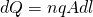The moving charges are equivalent to this total charge that we calculated right now. That way by substituting dQ as q in the magnetic field equation we get: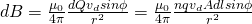The component: nqvdA is of course equal to the current I that flows in the section of length dl which means that we can re-write the equation as:"magnitude" and vector forms...

The component dl is of course a vector that points in the trajectory where the infinitesmal current flows and so because of the cross product between dl and r (which is the normal to the area) we can clearly see that the magnetic field is again perpendicular to the current and section A. This means that the magnetic field will again be in a circular form around the wire, like we had with charges! To find the actual direction of the field lines we use the same right-hand rule that we had with charges, but this time the velocity is replaced by the current. This looks like this: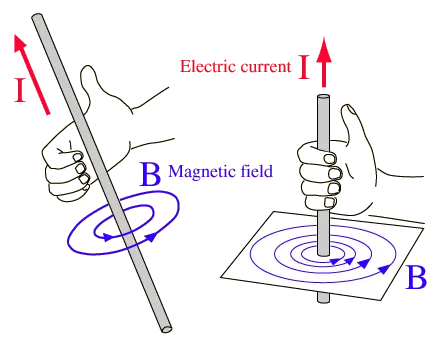Image source:## Biot-Savart law

By integrating this previous equation we end up with the so caleld Biot-Savart law which states: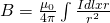When applying this law you should be cautious and should always check the following:

• The vector directions are very important. As already told before dl is always pointing in the direction of the current. The unit vector r is moving from dl to the points where we want to calculate the magnetic field (from the source to the observation point).
• The elementary charges and dB fields can have the same direction, which means that the total magnetic field is a simple sum of the magnitudes. But, these two vectors mostly have different directions which makes the integration much more difficult and takes us to an integral for each axis/component. For this second case symmetry is key and makes our calculations much much easier!
• Don't ever forget to use the superposition principle! Knowing the magnetic field of a simple case can sometimes help us when finding the magnetic field of another more complicated case.

So, yes this law applies to current-carrying conductors of a elementary length dl, what happens in other cases?

### Finite length

Suppose we have a straight current-carrying conductor of finite length 2a: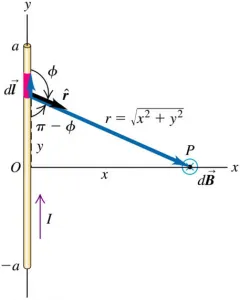By doing the calculations using Biot-Savart's and the Euclid theorem at the triangle we end up with: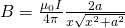where of course: r^2 = a^2 + b^2.

### Infinite length

Let's consider that the length of the conductor is infinite!

For a-> ∞ we get: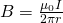where r is the distance of the point from the conductor/source.

As you can see, calculations where left out, cause we don't need them anyways now :P

## REFERENCES:

Mathematical equations that I had in this post where drawn using quicklatex!

### Electric fields:

Getting into Electromagnetism -> electromagnetim, electric charge, conductors, insulators, quantization

Coulomb's law with examples -> Coulomb's law, superposition principle, Coulomb constant, how to solve problems, examples

Electric fields and field lines -> Electric fields, Solving problems around Electric fields and field lines

Electric dipoles -> Electric dipole, torque, potential and field

Electric charge and field Exercises -> examples in electric charges and fields

### Electric flux:

Electric flux and Gauss's law -> Electric flux, Gauss's law

Applications of Gauss's law (part 1) -> applying Gauss's law, Gauss applications

Applications of Gauss's law (part 2) -> more Gauss applications

Electric flux exercises -> examples in electric flux and Gauss's law

### Electric potential:

Electric potential energy -> explanation of work-energy, electric potential energy

Calculating electric potentials -> more stuff about potential energy, potential, calculating potentials

Millikan's Oil Drop Experiment -> Millikan's experiment, electronvolt

Cathode ray tubes explained using electric potential -> cathode ray tube explanation

Electric potential exercises (part 1) -> applications of potential

Electric potential exercises (part 2) -> applications of potential gradient, advanced examples

### Capacitance:

Capacitors (Condensers) and Capacitance -> Capacitors, capacitance, calculating capacitance

How to solve problems around Capacitors -> combination, solving problems, simple example

Electric field energy and density -> Electric field energy, energy density

Dielectric materials -> Dielectrics, dielectric constant, permittivity and strength, how to solve problems

Electric capacitance exercises -> examples in capacitance, energy density and dielectrics

### Current, resistance and EMF:

Electric current -> Electric current, current density

Electrical resistivity and conductivity -> Electrical resistivity, conductivity, thermal coefficient of resistivity, hyperconductivity

Electric resistance -> Resistance, temperature, resistors

Electromotive Force (EMF) and Internal resistance -> Electromotive force, internal resistance

Power and Wattage of Electronic Circuits -> Power in general, power/wattage of electronic circuits

Electric current, resistance and emf exercises -> exampes in all those topics

### Direct current (DC) circuits:

Resistor Combinations -> Resistor combinations, how to solve problems

Kirchhoff's laws with applications -> Kirchhoff's laws, how to solve problems, applications

Electrical measuring instruments -> what are they?, types list, getting into some of them, an application

Electronic circuits with resistors and capacitors (R-C) -> R-C Circuit, charging, time constant, discharging, how to apply

RC circuit exercises -> examples in Kirchhoff, charging, discharging capacitor with/without internal resistance

### Magnetic field and forces:

Magnetic fields -> Magnetism, Magnetic field

Magnetic field lines and Gauss's law of Magnetism -> magnetic field lines, mono- and dipoles, Flux, Gauss's law of magnetism

The motion of charged particles inside of a magnetic field -> straight-line, spiral and helical particle motion

Applications of charged particle motion -> CERN, Cyclotrons, Synchrotrons, Cavity Magetron, Mass Spectrometry and Magnetic lens

Magnetic force applied on Current-Carrying Conductors -> magnetic force on current-carrying conductors/wires, proofs

Magnetic force and torque applied on current loops (circuits) -> magnetic force on current loops, magnetic moment and torque

Explaining the Physics behind Electromotors -> tesla, history and explaining the physics behind them

Magnetic field exercises -> examples in magnetic force, magnetic flux, particle motion and forces/torque on current-carrying conductors

### Magnetic field sources:

Magnetic field of a moving charged particle -> moving charge, magnetic field, force between parallel charged particles

And this is actually it for today's post! Next time I'm thinking of getting into the force between two parallel conductors and maybe even the magnetic field of current loops...

Bye!

H2
H3
H4
3 columns
2 columns
1 column